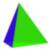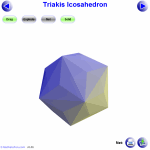# Polyhedrons

A polyhedron is a solid with flat faces
(from Greek poly- meaning "many" and -hedron meaning "face").

Each face is a polygon (a flat shape with straight sides).

## Examples of Polyhedra:Cube
Its faces are all squaresTriangular Prism
Its faces are triangles
and rectanglesDodecahedron
What faces does it have?

So no curved surfaces: cones, spheres and cylinders are not polyhedrons.

## Common PolyhedraPlatonic SolidsPrismsPyramids

Note: the plural of polyhedron is either polyhedrons or polyhedra

## Many MoreExplore 100s of Animated Polyhedron Models. You can also see some Images of Polyhedra if you want.

## Counting Faces, Vertices and Edges

When we count the number of faces (the flat surfaces), vertices (corner points), and edges of a polyhedron we discover an interesting thing:

The number of faces
plus the number of vertices
minus the number of edges equals 2

This can be written neatly as a little equation:

### F + V − E = 2

It is known as Euler's Formula (or the "Polyhedral Formula") and is very useful to make sure we have counted correctly!### Example: Cube

A cube has:

• 6 Faces
• 8 Vertices (corner points)
• 12 Edges

F + V − E = 6 + 8 − 12 = 2### Example: Triangular Prism

This prism has:

• 5 Faces
• 6 Vertices (corner points)
• 9 Edges

F + V − E = 5 + 6 − 9 = 2

But there are cases where it does not work! Read Euler's Formula for more.## Diagonals

A diagonal is a straight line inside a shape that goes from one corner to another (but not an edge).

A polyhedron can have lots of diagonals. Can you think of one without diagonals?